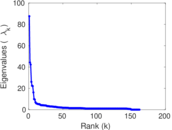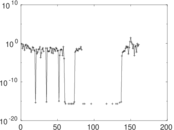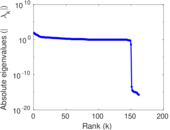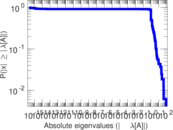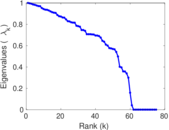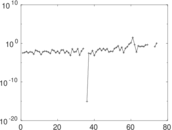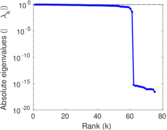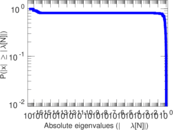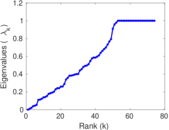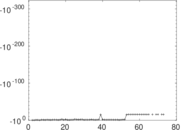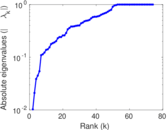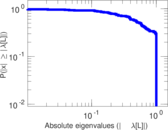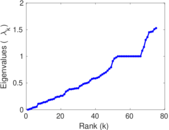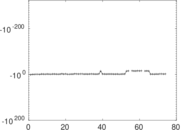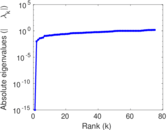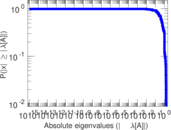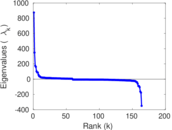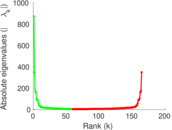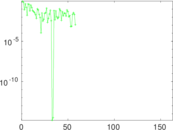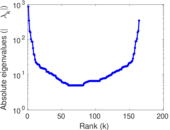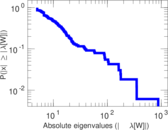# Wikibooks edits (ia)

This is the bipartite edit network of the Interlingua Wikibooks. It contains users and pages from the Interlingua Wikibooks, connected by edit events. Each edge represents an edit. The dataset includes the timestamp of each edit.

 Code `bia` Internal name `edit-iawikibooks` Name Wikibooks edits (ia) Data source http://dumps.wikimedia.org/ AvailabilityDataset is available for download Consistency checkDataset passed all tests Category Authorship network Dataset timestamp 2017-10-20 Node meaning User, article Edge meaning Edit Network formatBipartite, undirected Edge typeUnweighted, multiple edges Temporal dataEdges are annotated with timestamps

## Statistics

 Size n = 885 Left size n1 = 164 Right size n2 = 721 Volume m = 1,494 Unique edge count m̿ = 899 Wedge count s = 35,960 Claw count z = 1,805,315 Cross count x = 73,363,027 Square count q = 1,052 4-Tour count T4 = 154,830 Maximum degree dmax = 319 Maximum left degree d1max = 319 Maximum right degree d2max = 126 Average degree d = 3.376 27 Average left degree d1 = 9.109 76 Average right degree d2 = 2.072 12 Fill p = 0.007 602 92 Average edge multiplicity m̃ = 1.661 85 Size of LCC N = 636 Diameter δ = 14 50-Percentile effective diameter δ0.5 = 5.077 01 90-Percentile effective diameter δ0.9 = 7.397 32 Median distance δM = 6 Mean distance δm = 5.041 60 Gini coefficient G = 0.671 959 Balanced inequality ratio P = 0.238 621 Left balanced inequality ratio P1 = 0.166 667 Right balanced inequality ratio P2 = 0.320 616 Relative edge distribution entropy Her = 0.836 997 Power law exponent γ = 5.009 28 Tail power law exponent γt = 2.621 00 Tail power law exponent with p γ3 = 2.621 00 p-value p = 0.000 00 Left tail power law exponent with p γ3,1 = 1.921 00 Left p-value p1 = 0.062 000 0 Right tail power law exponent with p γ3,2 = 4.781 00 Right p-value p2 = 0.006 000 00 Degree assortativity ρ = −0.217 884 Degree assortativity p-value pρ = 4.021 66 × 10−11 Spectral norm α = 87.561 1 Algebraic connectivity a = 0.010 256 9 Spectral separation |λ1[A] / λ2[A]| = 1.981 35 Controllability C = 575 Relative controllability Cr = 0.657 143

## Plots

### Fruchterman–Reingold graph drawing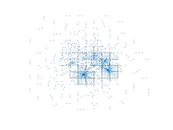### Degree distribution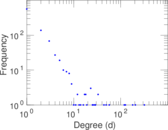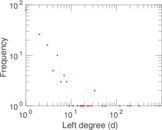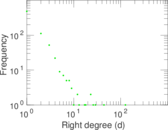### Cumulative degree distribution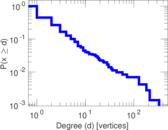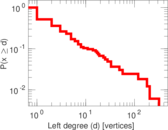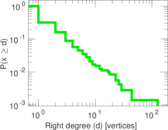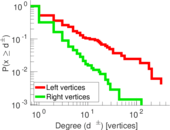### Lorenz curve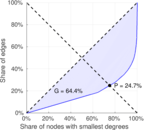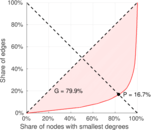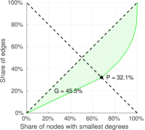### Spectral distribution of the adjacency matrix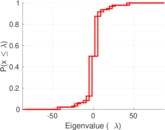### Spectral distribution of the normalized adjacency matrix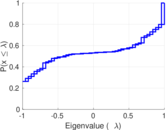### Spectral distribution of the Laplacian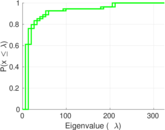### Spectral graph drawing based on the adjacency matrix### Spectral graph drawing based on the Laplacian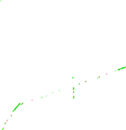### Spectral graph drawing based on the normalized adjacency matrix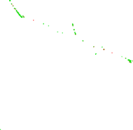### Degree assortativity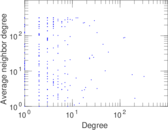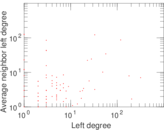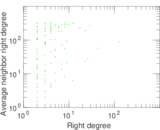### Zipf plot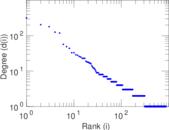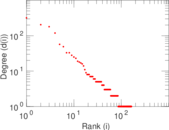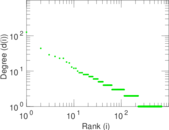### Hop distribution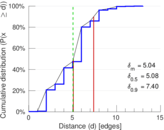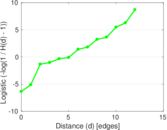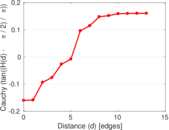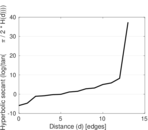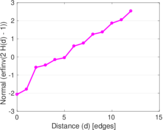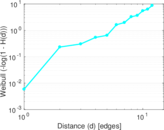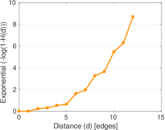### Double Laplacian graph drawing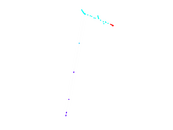### Delaunay graph drawing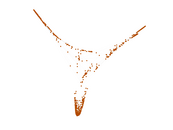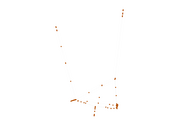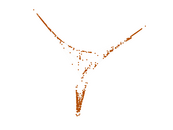### Edge weight/multiplicity distribution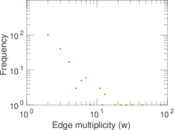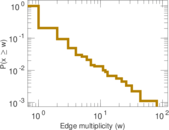### Temporal distribution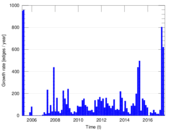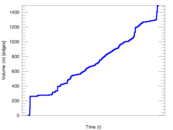### Temporal hop distribution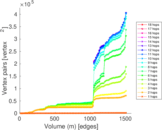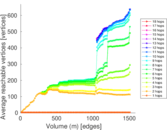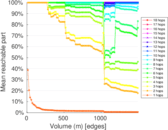### Diameter/density evolution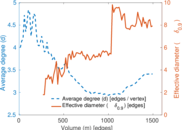### Matrix decompositions plots